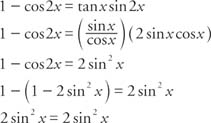## Double‐Angle and Half‐Angle Identities

Special cases of the sum and difference formulas for sine and cosine yields what is known as the double‐angle identities and the half‐angle identities. First, using the sum identity for the sine,

sin 2α = sin (α + α)

sin 2α = sin α cos α + cos α sin α

sin 2α = 2 sin α cos α

Similarly for the cosine,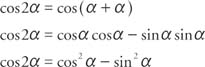Using the Pythagorean identity, sin 2 α+cos 2α=1, two additional cosine identities can be derived.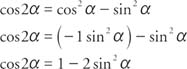andThe half‐angle identities for the sine and cosine are derived from two of the cosine identities described earlier.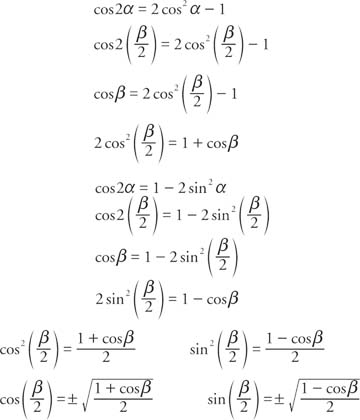The sign of the two preceding functions depends on the quadrant in which the resulting angle is located.

Example 1: Find the exact value for sin 105° using the half‐angle identity.

In the following verification, remember that 105° is in the second quadrant, and sine functions in the second quadrant are positive. Also, 210° is in the third quadrant, and cosine functions in the third quadrant are negative. From Figure  1, the reference triangle of 210° in the third quadrant is a 30°–60°–90° triangle. Therefore, cos 210° = −cos 30°.Figure  1
Drawing for Example 1.

Using the half‐angle identity for sine,Example 2: Find the exact value for cos 165° using the half‐angle identity.

In the following verification, remember that 165° is in the second quadrant, and cosine functions in the second quadrant are negative. Also, 330° is in the fourth quadrant, and cosine functions in the fourth quadrant are positive. From Figure 2 , the reference triangle of 330° in the fourth quadrant is a 30°–60°–90° triangle. Therefore, cos 330° = cos 30°.Figure  2
Drawing for Example 2.

Using the half‐angle identity for the cosine,Example 3: Use the double‐angle identity to find the exact value for cos 2 x given that sin x =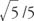.

Because sin x is positive, angle x must be in the first or second quadrant. The sign of cos 2 x will depend on the size of angle x. If 0° < x < 45° or 135° < x < 180°, then 2 x will be in the first or fourth quadrant and cos2 x will be positive. On the other hand, if 45° < x < 90° or 90° < x < 135”, then 2 x will be in the second or third quadrant and cos 2 x will be negative.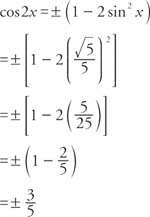Example 4: Verify the identity 1 − cos 2 x = tan x sin 2 x.